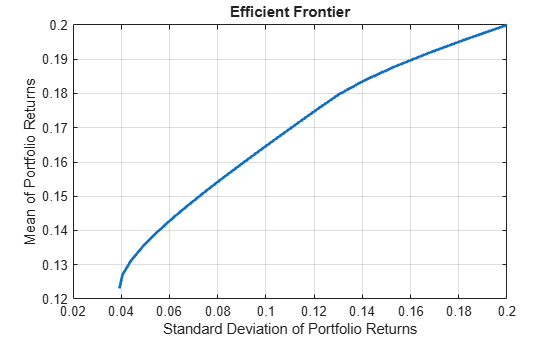# portopt

Portfolios on constrained efficient frontier

`portopt` has been partially removed and will no longer accept `ConSet` or `varargin` arguments. Use `Portfolio` instead to solve portfolio problems that are more than a long-only fully-invested portfolio. For information on the workflow when using `Portfolio` objects, see Portfolio Object Workflow. For more information on migrating `portopt` code to `Portfolio`, see portopt Migration to Portfolio Object.

## Syntax

``[PortRisk,PortReturn,PortWts] = portopt(ExpReturn,ExpCovariance)``
``[PortRisk,PortReturn,PortWts] = portopt(___,NumPorts,PortReturn)``
``portopt(___,NumPorts,PortReturn)``

## Description

example

````[PortRisk,PortReturn,PortWts] = portopt(ExpReturn,ExpCovariance)` sets up the most basic portfolio problem with weights greater than or equal to `0` that must sum to `1`. All that is necessary to solve this problem is the mean and covariance of asset returns. By default, `portopt` returns 10 equally-spaced points on the efficient frontier. `portopt` solves the "standard" mean-variance portfolio optimization problem for a long-only fully-invested investor with no additional constraints. Specifically, every portfolios on the efficient frontier has non-negative weights that sum to 1.```

example

````[PortRisk,PortReturn,PortWts] = portopt(___,NumPorts,PortReturn)` specifies options using one or more optional arguments in addition to the input arguments in the previous syntax.```

example

````portopt(___,NumPorts,PortReturn)` returns a plot of the efficient frontier if `portopt` is invoked with no output arguments.```

## Examples

collapse all

Use `portopt` to connect 20 portfolios along the efficient frontier having evenly spaced returns. By default, choose among portfolios without short-selling and scale the value of the portfolio to 1.

```ExpReturn = [0.1 0.2 0.15]; ExpCovariance = [0.005 -0.010 0.004 -0.010 0.040 -0.002 0.004 -0.002 0.023]; NumPorts = 20; portopt(ExpReturn, ExpCovariance, NumPorts)```## Input Arguments

collapse all

Expected (mean) return of each asset, specified as a `1`-by-number of assets (`NASSETS`) vector.

Data Types: `double`

Covariance of the asset returns, specified as a `NASSETS`-by-`NASSETS` matrix.

Data Types: `double`

(Optional) Number of portfolios generated along the efficient frontier, specified as a scalar numeric. Returns are equally spaced between the maximum possible return and the minimum risk point. If `NumPorts` is empty (entered as `[]`), `portopt` computes 10 equally spaced points. If you specify `1`, `portopt` returns the minimum-risk portfolio.

Note

If not over-ridden by `PortReturn`, these portfolios are spaced evenly from the minimum to the maximum return on the efficient frontier. If `NumPorts` = 1, then the minimum-risk portfolio is computed (positive integer).

Data Types: `double`

(Optional) Target portfolio returns to be computed on the efficient frontier, specified as a number of portfolios (`NPORTS`-by-`1` vector). If not entered or empty, `NumPorts` equally spaced returns between the minimum and maximum possible values are used.

Note

`portopt` requires that if you set `PortReturn`, `NumPorts` should be empty. If you specify `PortReturn` with a nonempty vector, `PortReturn` overrides `NumPorts`. If any returns in `PortReturn` fall outside the range of returns on the efficient frontier, `portopt` generates a warning and the efficient portfolios closest to the endpoints of the efficient frontier are computed.

Data Types: `double`

## Output Arguments

collapse all

Standard deviation of each portfolio, returned as a `NPORTS`-by-`1` vector.

`PortWts` is an `NPORTS`-by-`NASSETS` matrix of weights allocated to each asset. Each row represents a portfolio. The total of all weights in a portfolio is 1.

Expected return of each portfolio, returned as a `NPORTS`-by-`1` vector.

Weights allocated to each asset, returned as a `NPORTS`-by-`NASSETS` matrix. Each row represents a portfolio. The total of all weights in a portfolio is 1.

## Version History

Introduced before R2006a